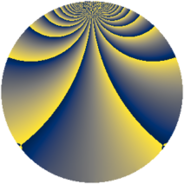# Properties

 Label 693.2.cqLevel $693$ Weight $2$ Character orbit 693.cq Rep. character $\chi_{693}(29,\cdot)$ Character field $\Q(\zeta_{30})$ Dimension $576$ Newform subspaces $1$ Sturm bound $192$ Trace bound $0$

# Related objects

## Defining parameters

 Level: $$N$$ $$=$$ $$693 = 3^{2} \cdot 7 \cdot 11$$ Weight: $$k$$ $$=$$ $$2$$ Character orbit: $$[\chi]$$ $$=$$ 693.cq (of order $$30$$ and degree $$8$$) Character conductor: $$\operatorname{cond}(\chi)$$ $$=$$ $$99$$ Character field: $$\Q(\zeta_{30})$$ Newform subspaces: $$1$$ Sturm bound: $$192$$ Trace bound: $$0$$

## Dimensions

The following table gives the dimensions of various subspaces of $$M_{2}(693, [\chi])$$.

Total New Old
Modular forms 800 576 224
Cusp forms 736 576 160
Eisenstein series 64 0 64

## Trace form

 $$576q - 6q^{3} + 72q^{4} - 6q^{5} + 10q^{6} + 2q^{9} + O(q^{10})$$ $$576q - 6q^{3} + 72q^{4} - 6q^{5} + 10q^{6} + 2q^{9} - 12q^{11} + 56q^{12} - 6q^{15} + 72q^{16} - 20q^{18} + 60q^{19} + 24q^{20} + 6q^{22} - 30q^{24} - 78q^{25} - 90q^{29} - 60q^{30} + 6q^{31} + 2q^{33} + 12q^{34} - 26q^{36} - 12q^{37} - 120q^{38} - 110q^{39} + 24q^{45} + 24q^{47} + 12q^{48} - 72q^{49} + 30q^{51} - 60q^{57} - 120q^{59} + 32q^{60} - 144q^{64} + 20q^{66} - 36q^{67} - 390q^{68} - 26q^{69} - 150q^{72} - 32q^{75} - 232q^{78} + 106q^{81} - 36q^{82} + 90q^{83} + 80q^{84} + 102q^{86} + 114q^{88} + 200q^{90} - 36q^{91} + 228q^{92} + 66q^{93} + 120q^{95} + 320q^{96} - 60q^{97} - 158q^{99} + O(q^{100})$$

## Decomposition of $$S_{2}^{\mathrm{new}}(693, [\chi])$$ into newform subspaces

Label Dim. $$A$$ Field CM Traces $q$-expansion
$$a_2$$ $$a_3$$ $$a_5$$ $$a_7$$
693.2.cq.a $$576$$ $$5.534$$ None $$0$$ $$-6$$ $$-6$$ $$0$$

## Decomposition of $$S_{2}^{\mathrm{old}}(693, [\chi])$$ into lower level spaces

$$S_{2}^{\mathrm{old}}(693, [\chi]) \cong$$ $$S_{2}^{\mathrm{new}}(99, [\chi])$$$$^{\oplus 2}$$